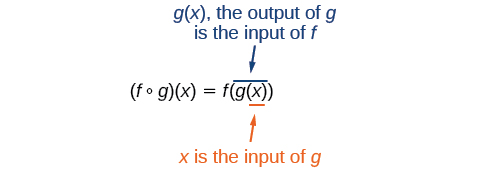# 1.4 Composition of functions  (Page 2/9)

 Page 2 / 9

## Performing algebraic operations on functions

Find and simplify the functions $\text{\hspace{0.17em}}\left(g-f\right)\left(x\right)\text{\hspace{0.17em}}$ and $\text{\hspace{0.17em}}\left(\frac{g}{f}\right)\left(x\right),\text{\hspace{0.17em}}$ given $\text{\hspace{0.17em}}f\left(x\right)=x-1\text{\hspace{0.17em}}$ and $\text{\hspace{0.17em}}g\left(x\right)={x}^{2}-1.\text{\hspace{0.17em}}$ Are they the same function?

Begin by writing the general form, and then substitute the given functions.

No, the functions are not the same.

Note: For $\text{\hspace{0.17em}}\left(\frac{g}{f}\right)\left(x\right),\text{\hspace{0.17em}}$ the condition $\text{\hspace{0.17em}}x\ne 1\text{\hspace{0.17em}}$ is necessary because when $\text{\hspace{0.17em}}x=1,\text{\hspace{0.17em}}$ the denominator is equal to 0, which makes the function undefined.

Find and simplify the functions $\text{\hspace{0.17em}}\left(fg\right)\left(x\right)\text{\hspace{0.17em}}$ and $\text{\hspace{0.17em}}\left(f-g\right)\left(x\right).$

Are they the same function?

$\begin{array}{l}\left(fg\right)\left(x\right)=f\left(x\right)g\left(x\right)=\left(x-1\right)\left({x}^{2}-1\right)={x}^{3}-{x}^{2}-x+1\\ \left(f-g\right)\left(x\right)=f\left(x\right)-g\left(x\right)=\left(x-1\right)-\left({x}^{2}-1\right)=x-{x}^{2}\end{array}$

No, the functions are not the same.

## Create a function by composition of functions

Performing algebraic operations on functions combines them into a new function, but we can also create functions by composing functions. When we wanted to compute a heating cost from a day of the year, we created a new function that takes a day as input and yields a cost as output. The process of combining functions so that the output of one function becomes the input of another is known as a composition of functions . The resulting function is known as a composite function . We represent this combination by the following notation:

$\left(f\circ g\right)\left(x\right)=f\left(g\left(x\right)\right)$

We read the left-hand side as $“f\text{\hspace{0.17em}}$ composed with $\text{\hspace{0.17em}}g\text{\hspace{0.17em}}$ at $\text{\hspace{0.17em}}x,”$ and the right-hand side as $“f\text{\hspace{0.17em}}$ of $\text{\hspace{0.17em}}g\text{\hspace{0.17em}}$ of $\text{\hspace{0.17em}}x.”$ The two sides of the equation have the same mathematical meaning and are equal. The open circle symbol $\text{\hspace{0.17em}}\circ \text{\hspace{0.17em}}$ is called the composition operator. We use this operator mainly when we wish to emphasize the relationship between the functions themselves without referring to any particular input value. Composition is a binary operation that takes two functions and forms a new function, much as addition or multiplication takes two numbers and gives a new number. However, it is important not to confuse function composition with multiplication because, as we learned above, in most cases $f\left(g\left(x\right)\right)\ne f\left(x\right)g\left(x\right).$

It is also important to understand the order of operations in evaluating a composite function. We follow the usual convention with parentheses by starting with the innermost parentheses first, and then working to the outside. In the equation above, the function $\text{\hspace{0.17em}}g\text{\hspace{0.17em}}$ takes the input $\text{\hspace{0.17em}}x\text{\hspace{0.17em}}$ first and yields an output $\text{\hspace{0.17em}}g\left(x\right).\text{\hspace{0.17em}}$ Then the function $\text{\hspace{0.17em}}f\text{\hspace{0.17em}}$ takes $\text{\hspace{0.17em}}g\left(x\right)\text{\hspace{0.17em}}$ as an input and yields an output $\text{\hspace{0.17em}}f\left(g\left(x\right)\right).$In general, $\text{\hspace{0.17em}}f\circ g\text{\hspace{0.17em}}$ and $\text{\hspace{0.17em}}g\circ f\text{\hspace{0.17em}}$ are different functions. In other words, in many cases $\text{\hspace{0.17em}}f\left(g\left(x\right)\right)\ne g\left(f\left(x\right)\right)\text{\hspace{0.17em}}$ for all $\text{\hspace{0.17em}}x.\text{\hspace{0.17em}}$ We will also see that sometimes two functions can be composed only in one specific order.

For example, if $\text{\hspace{0.17em}}f\left(x\right)={x}^{2}\text{\hspace{0.17em}}$ and $\text{\hspace{0.17em}}g\left(x\right)=x+2,$ then

but

These expressions are not equal for all values of $\text{\hspace{0.17em}}x,\text{\hspace{0.17em}}$ so the two functions are not equal. It is irrelevant that the expressions happen to be equal for the single input value $\text{\hspace{0.17em}}x=-\frac{1}{2}.$

Note that the range of the inside function (the first function to be evaluated) needs to be within the domain of the outside function. Less formally, the composition has to make sense in terms of inputs and outputs.

#### Questions & Answers

how fast can i understand functions without much difficulty
Joe Reply
what is set?
Kelvin Reply
a colony of bacteria is growing exponentially doubling in size every 100 minutes. how much minutes will it take for the colony of bacteria to triple in size
Divya Reply
I got 300 minutes. is it right?
Patience
no. should be about 150 minutes.
Jason
It should be 158.5 minutes.
Mr
ok, thanks
Patience
100•3=300 300=50•2^x 6=2^x x=log_2(6) =2.5849625 so, 300=50•2^2.5849625 and, so, the # of bacteria will double every (100•2.5849625) = 258.49625 minutes
Thomas
what is the importance knowing the graph of circular functions?
Arabella Reply
can get some help basic precalculus
ismail Reply
What do you need help with?
Andrew
how to convert general to standard form with not perfect trinomial
Camalia Reply
can get some help inverse function
ismail
Rectangle coordinate
Asma Reply
how to find for x
Jhon Reply
it depends on the equation
Robert
yeah, it does. why do we attempt to gain all of them one side or the other?
Melissa
whats a domain
mike Reply
The domain of a function is the set of all input on which the function is defined. For example all real numbers are the Domain of any Polynomial function.
Spiro
Spiro; thanks for putting it out there like that, 😁
Melissa
foci (–7,–17) and (–7,17), the absolute value of the differenceof the distances of any point from the foci is 24.
Churlene Reply
difference between calculus and pre calculus?
Asma Reply
give me an example of a problem so that I can practice answering
Jenefa Reply
x³+y³+z³=42
Robert
dont forget the cube in each variable ;)
Robert
of she solves that, well ... then she has a lot of computational force under her command ....
Walter
what is a function?
CJ Reply
I want to learn about the law of exponent
Quera Reply
explain this
Hinderson Reply

### Read also:

#### Get Jobilize Job Search Mobile App in your pocket Now!

Source:  OpenStax, Precalculus. OpenStax CNX. Jan 19, 2016 Download for free at https://legacy.cnx.org/content/col11667/1.6
Google Play and the Google Play logo are trademarks of Google Inc.

Notification Switch

Would you like to follow the 'Precalculus' conversation and receive update notifications?ByByBy OpenStaxBy OpenStaxBy Dionne MahaffeyBy Melinda SalzerBy OpenStaxBy John GabrieliBy Subramanian DivyaBy Stephen VoronBy OpenStaxBy OpenStax